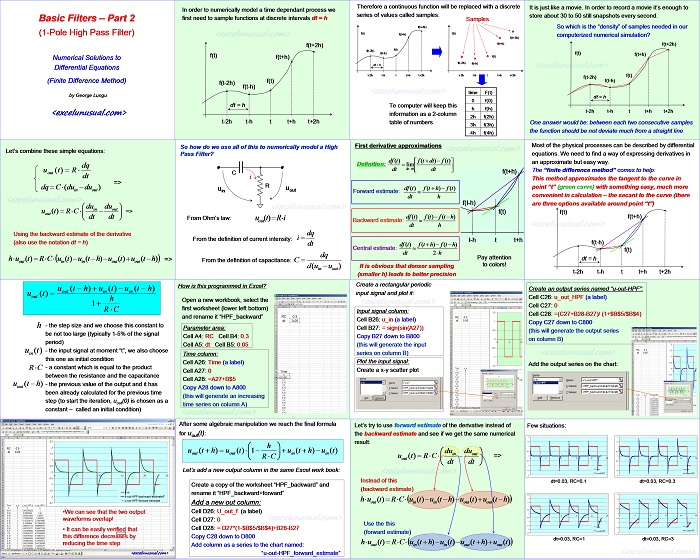# Introduction to Numerical Methods – Basic Filters #2

This post shows the steps for building a first model of a single pole RC high pass filter (HPF) in Excel based on numerical solution using the finite difference method.

It is quite elementary to understand, try to go through the proof regardless of your background.[sociallocker][/sociallocker]

## Basic Filters – Part 2 (1-Pole High Pass Filter)

Numerical Solutions to Differential Equations (Finite Difference Method)

by George Lungu

In order to numerically model a time dependant process we
first need to sample functions at discrete intervals dt = h

f(t+2h)
f(t)
f(t+h)
f(t)
f(t-2h)
f(t-h)
dt = h
t-2h t-h t t+h t+2h

Therefore a continuous function will be replaced with a discrete series of values called samples.

Samples
f(t+2h)
f(t) f(t)
f(t+h)
f(t+2h)
f(t+h)
f(t)
f(t-2h)
f(t-h)
f(t)
f(t-2h)
f(t-h)
dt = h
t-2h t-h t t+h t+2h t-2h t-h t t+h t+2h
time F(t)
0 f(0)
Te computer will keep this information as a 2-column table of numbers.

It is just like a movie. In order to record a movie it’s enough to store about 30 to 50 still snapshots every second.
So which is the “density” of samples needed in our computerized numerical simulation?
f(t+2h)
f(t)
f(t+h)
f(t)
f(t-2h)
f(t-h)
dt = h
t-2h t-h t t+h t+2h

One answer would be: between each two consecutive samples the function should be not deviate much from a straight line.

Most of the physical processes can be described by differential equations. We need to find a way of expressing derivatives in an approximate but easy way.

### The “finite difference method” comes to help:

This method approximates the tangent to the curve in point “t” (green curve) with something easy, much more convenient in calculation – the secant to the curve (there are three options available around point “t”)
f(t)
f(t+h)
f(t-h)
f(t)
dt = h
t-2h t-h t t+h t+2h

#### First derivative approximations

Definition:  lim
Forward estimate: 

dt h
f(t-h)
f(t)
df (t ) f(t )* f (t – h)

Backward estimate:

dt h
t-2h t-h t t+h
df (t) f(t-h)* f (t -h)

Central estimate:
dt 2* h

Pay attention to colors!

It is obvious that denser sampling (smaller h) leads to better precision

So how do we use all of this to numerically model a High Pass Filter?
C
i
R
uout
uin

From Ohm’s law: uout(t )  R  i
dq
i

From the definition of current intensity:
dt
dq

From the definition of capacitance: C
d(u u )
in out

Let’s combine these simple equations:
dq

out(u t)* R
dt
=>

Using the backward estimate of the derivative (also use the notation dt = h)

h – the step size and we choose this constant to be not too large (typically 1-5% of the signal period)
– the input signal at moment “t”, we also choose
u (t )
in
this one as initial condition

– a constant which is equal to the product between the resistance and the capacitance

– the previous value of the output and it has  been already calculated for the previous time step (to start the iteration, uout(0) is chosen as a constant – called an initial condition)

### How is this programmed in Excel?

Open a new workbook, select the first worksheet (lower left bottom) and rename it “HPF_backward”

Parameter area:
Cell A4: RC Cell B4: 0.3
Cell A5: dt Cell B5: 0.05

Time column:
Cell A26: Time (a label)
Cell A27: 0
Cell A28: =A27+B\$5
Copy A28 down to A800

(this will generate an increasing time series on column A)

Create a rectangular periodic input signal and plot it:

Input signal column:
Cell B26: u_in (a label)
Cell B27: = sign(sin(A27))
Copy B27 down to B800

(this will generate the input series on column B)

Plot the input signal:

Create a x-y scatter plot

Create an output series named “u-out-HPF”:
Cell C26: u_out_HPF (a label)
Cell C27: 0
Cell C28: =(C27+B28-B27)/ (1+\$B\$5/\$B\$4)
Copy C27 down to C800

(this will generate the output series on column B)

Add the output series on the chart:

A few situations:

0.5 0.5
0 0
0 5 10 15 20 25 0 5 10 15 20 25
u-out-HPF u-out-HPF
dt=0.03, RC=0.1 dt=0.03, RC=0.3

0 5 10 15 20 25 0 5 10 15 20 25

u-in u-out
u-out-HPF u-out-HPF
dt=0.03, RC=1 dt=0.03, RC=3

Let’s try to use forward estimate of the derivative instead of the backward estimate and see if we get the same numerical
result:

(backward estimate)
h-u (t)* R*C- u (t)+u (t+h)*u (t)*u (t-h)
out in in out out

Use the this (forward estimate)

After some algebraic manipulation we reach the final formula for uout(t):

Let’s add a new output column in the same Excel work book:

Create a copy of the worksheet “HPF_backward” and rename it “HPF_backward+forward”

Cell D26: U_out_f (a label)
Cell D27: 0
Cell D28: = D27*(1-\$B\$5/\$B\$4)+B28-B27
Copy C28 down to D800

Add column as a series to the chart named:

“u-out-HPF_forward_estimate”

•We can see that the two output waveforms overlap!
• It can be easily verified that this difference decreases by reducing the time step

by George Lungu <excelunusual.com>